#Basic DefinitionsIt is an amazing fact that by adjoining the imaginary unit i to the real numbers we obtain a complete number field called `` The Complex Numbers." In this amazing number field every algebraic equation in z with complex coefficients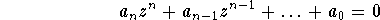has a solution. To prove this fact we need Liouville's Theorem, but to get started using complex numbers all we need are the following basic rules.

# Rules of Complex Arithmetic

1.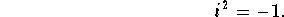2. Every complex number has the ``Standard Form''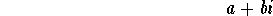for some real a and b.

3. For real a and b,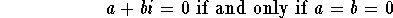4.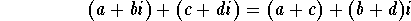5.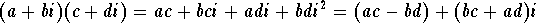Click on EXERCISES for some practice using these rules.

## Division

Notice that rules 4 and 5 state that we can't get out of the complex numbers by adding (or subtracting) or multiplying two complex numbers together. What about dividing one complex number by another? Is the result another complex number? Let's ask the question in another way. If you are given four real numbers a,b,c and d, can you find two other real numbers x and y so that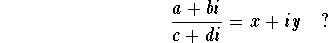As an exercise in using rules 1 through 5, multiply both sides of the above equation by c + di and then solve for x and y to prove that the answer to our question is yes.( Click on help for a solution.)

OK, so we can divide by c + di if c and d are not both zero. But there is a much easier way to do division.

Notice that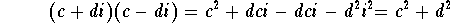We say that c+di and c-di are complex conjugates. To simplify a complex fraction, multiply the numerator and the denominator by the complex conjugate of the denominator.

## Examples of division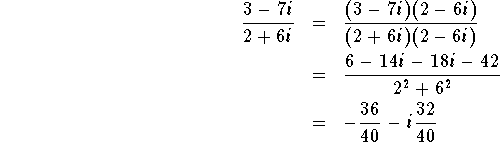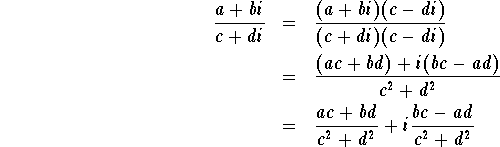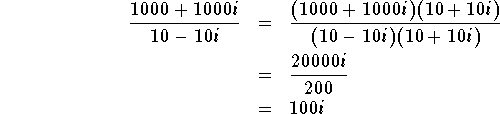## Real and Imaginary Parts

If z= a+bi is a complex number and a and b are real, we say that a is the real part of z and that b is the imaginary part of z and we write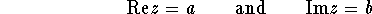Exercise:

Find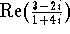and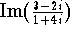. (Solution)

## Complex Conjugates

If z=a +bi is a complex number with real part a and imaginary part b, then we denote the complex conjugate of z by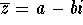.

Exercise:

Write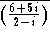in standard form.

Exercise:

Prove that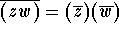for any pair of complex numbers and similarly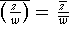.

Exercise:

Prove that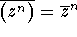for any integer n.

## Modulus of a Complex Number

The magnitude or modulus of a complex number z is denoted |z| and defined as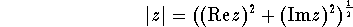Notice that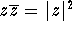. (proof.)

Exercise:

Prove that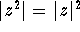. (Solution)[Algebra] [Complex Variables]
[Geometry] [Trigonometry ]
[Calculus] [Differential Equations] [Matrix Algebra]S.O.S MATHematics home page

Do you need more help? Please post your question on our S.O.S. Mathematics CyberBoard.Author: Michael O'Neill